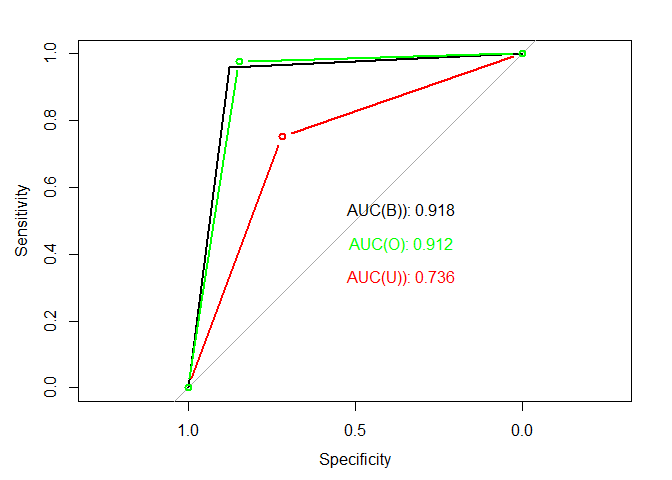In today’s lab session we will explore data about processed by travel agency claims. Based on this data we will try to predict either claim will be accepted or rejected. We will use various metrics to measure the performance of our data and in case they are not satisfying, we will try to improve our model using various techniques.

Let’s install & load the required packages:

install.packages("tidyverse")
library("tidyverse")

library("mice") # for imputation

library("randomForest")
library("rfUtilities") # for Cross-Validation

library("ROSE") # for balancing

library("pROC") # for ROC and AUC

Our dataset contains details about the claim, which we will use to predict their status (approved or not).

travel_insurance <- read.csv(file.choose()) # travel_insurance.csv
View(travel_insurance)
• Target: Claim Status (Claim)
• Name of agency (Agency)
• Type of travel insurance agencies (Agency.Type)
• Distribution channel of travel insurance agencies (Distribution.Channel)
• Name of the travel insurance products (Product.Name)
• Duration of travel (Duration)
• Destination of travel (Destination)
• Amount of sales of travel insurance policies (Net.Sales)
• Commission received for travel insurance agency (Commission)
• Gender of insured (Gender)
• Age of insured (Age)

## Data exploration

str(travel_insurance)
## 'data.frame':    19659 obs. of  11 variables:
##  $Agency : Factor w/ 16 levels "ADM","ART","C2B",..: 4 7 7 7 10 7 10 3 3 7 ... ##$ Agency.Type         : Factor w/ 2 levels "Airlines","Travel Agency": 2 2 2 2 1 2 1 1 1 2 ...
##  $Distribution.Channel: Factor w/ 2 levels "Offline","Online": 1 2 2 2 2 2 2 2 2 2 ... ##$ Product.Name        : Factor w/ 25 levels "1 way Comprehensive Plan",..: 13 17 17 17 9 17 9 10 18 17 ...
##  $Claim : Factor w/ 2 levels "No","Yes": 1 1 1 1 1 1 1 2 1 1 ... ##$ Duration            : int  186 -1 60 47 33 6 3 12 190 29 ...
##  $Destination : Factor w/ 119 levels "ALBANIA","ARGENTINA",..: 67 3 3 112 57 25 67 95 95 112 ... ##$ Net.Sales           : num  -29 -49.5 -39.6 -39.6 -26 ...
##  $Commision..in.value.: num 9.57 29.7 23.76 23.76 9.1 ... ##$ Gender              : Factor w/ 3 levels "","F","M": 2 1 1 1 1 1 3 3 3 1 ...
travel_insurance[travel_insurance$Age > 114, "Age"] <- NA Now we can replace missing data with substituted values: m <- mice(travel_insurance, m=5) ## ## iter imp variable ## 1 1 Duration* Age ## 1 2 Duration* Age ## 1 3 Duration* Age ## 1 4 Duration* Age ## 1 5 Duration* Age ## 2 1 Duration* Age ## 2 2 Duration* Age ## 2 3 Duration* Age ## 2 4 Duration* Age ## 2 5 Duration* Age ## 3 1 Duration* Age ## 3 2 Duration* Age ## 3 3 Duration* Age ## 3 4 Duration* Age ## 3 5 Duration* Age ## 4 1 Duration* Age ## 4 2 Duration* Age ## 4 3 Duration* Age ## 4 4 Duration* Age ## 4 5 Duration* Age ## 5 1 Duration* Age ## 5 2 Duration* Age ## 5 3 Duration* Age ## 5 4 Duration* Age ## 5 5 Duration* Age ## * Please inspect the loggedEvents m2 <- complete(m, 1) Let’s check the values in dataset: <YOUR CODE> travel_insurance <- m2 Next, let’s study “Gender” column: <YOUR CODE> # calculate percenage for each value in column "Gender" <YOUR CODE> # plot to see the "Gender" variation As you can see, we have ~30% of the data where gender is defined. This give us 2 possible ways of dealing with the problem: • 1 - Remove all rows where Gender is NA (Leaves us ~30% of the current data); • 2 - Comeplitely exclude the feature from our model. In this case, we will pick the 2nd option. <YOUR CODE> # remove "Gender" Next, let’s deal with problem with big amount of factor levels in column “Destination”. First, let’s look at values: travel_insurance %>% group_by(Destination) %>% summarise(total=n()) %>% ggplot(aes(x=Destination,y=total))+ geom_bar(stat="identity")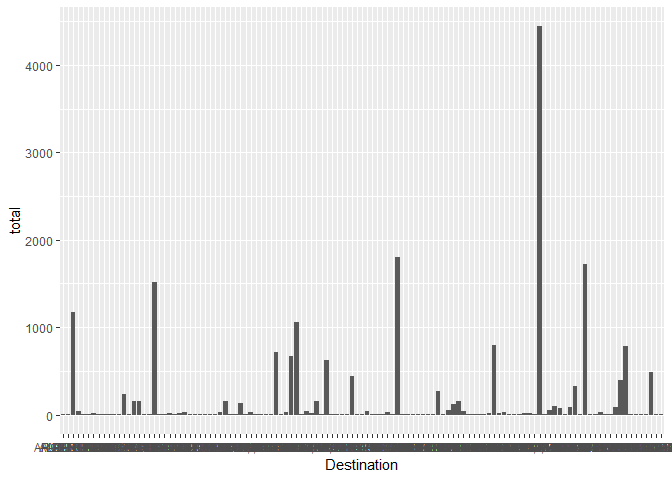Let’s convert destinations to numbers: travel_insurance_new <- travel_insurance %>% mutate(total_destination = ave(seq(nrow(travel_insurance)), Destination, FUN=length)) Next we define breaking points for creating bins. <YOUR CODE> # find breaking points <YOUR CODE> # create bins ## Building the model First, let’s create empty dataframe and function to add metrics in the dataframe, which we will use to compare the models: report <- data.frame(description = character(), accuracy = integer(), precision = integer(), recall = integer(), f1 = integer(), stringsAsFactors=FALSE) measures <- function(table, descr, df){ acc <- (table[1,1] + table[2,2]) / sum(table) prec <- table[2,2] / (table[2,2] + table[1,2]) rec <- table[2,2] / (table[2,1] + table[2,2]) f1 <- 2 * prec * rec / (prec + rec) print(paste("Accuracy: ", acc)) print(paste("Precision: ", prec)) print(paste("Recall: ", rec)) print(paste("F1 Score: ", f1)) tmpDf <- data.frame(descr, acc, prec, rec, f1) colnames(tmpDf) <- colnames(df) df <- rbind(df, tmpDf) return(df) } Next we separate the data for our model training. sample <- sample.int(n = nrow(travel_insurance_final), size = floor(0.7*nrow(travel_insurance_final)), replace = F) train <- travel_insurance_final[sample, ] test <- travel_insurance_final[-sample, ] ### Random forest Let’s try Random Forest algorithm: <YOUR CODE> # run Random Forest on train data <YOUR CODE> # predict the values Confusion matrix: cm_rf <- table(test_pred_rf, test$Claim)
cm_rf
##
## test_pred_rf   No  Yes
##          No  5614  272
##          Yes    7    5
report <- measures(cm_rf, "Random Forest", report)
##  "Accuracy:  0.952695829094608"
##  "Precision:  0.0180505415162455"
##  "Recall:  0.0180505415162455"
##  "F1 Score:  0.0180505415162455"

Let’s look at values in column “Claim”

ggplot(travel_insurance_final,aes(x=Claim, fill= Claim)) + geom_bar()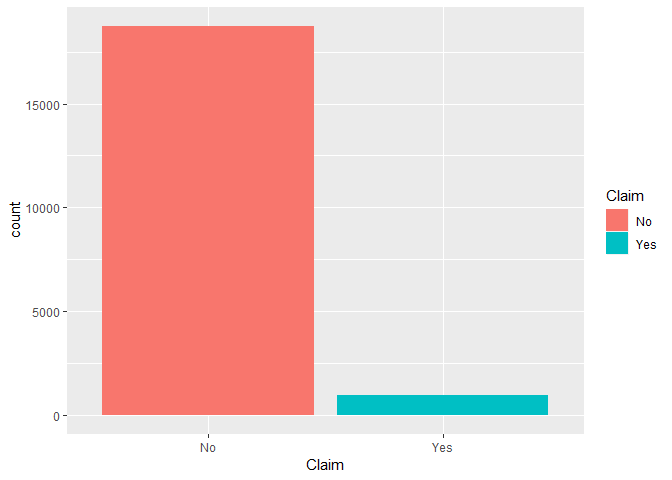# Imbalanced dataset

The data is imbalanced and thus we will use following techniques to balance it.

## Undersampling

travel_undersampling <- ovun.sample(Claim~. , data= travel_insurance_final, method = "under",
p=0.5, seed = 1000)$data ggplot(travel_undersampling,aes(x=Claim, fill= Claim)) + geom_bar()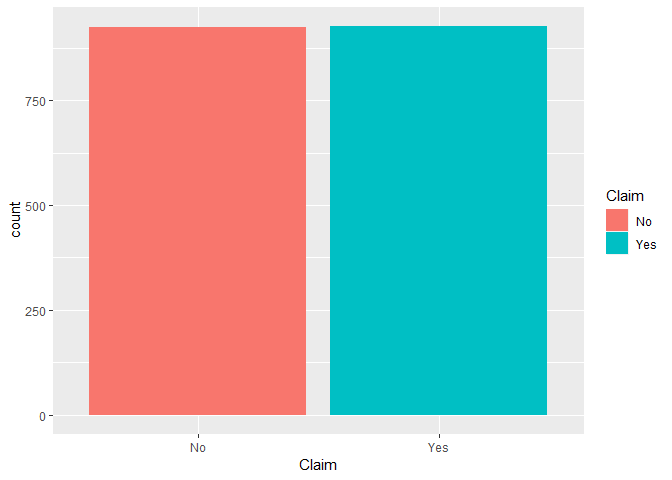### Random forest Data separation: sample <- sample.int(n = nrow(travel_undersampling), size = floor(0.7*nrow(travel_undersampling)), replace = F) train_u <- travel_undersampling[sample, ] test_u <- travel_undersampling[-sample, ] Training the model: rfModel_under <- randomForest(Claim ~., data = train_u) test_pred_rf_u <- predict(rfModel_under, newdata = test_u) Confusion matrix: cm_rf <- table(test_pred_rf_u, test_u$Claim)
cm_rf
##
## test_pred_rf_u  No Yes
##            No  199  69
##            Yes  78 210
report <- measures(cm_rf, "Random Forest. Undersampling", report)
##  "Accuracy:  0.735611510791367"
##  "Precision:  0.752688172043011"
##  "Recall:  0.752688172043011"
##  "F1 Score:  0.752688172043011"

## Oversampling

travel_oversampling <- ovun.sample(Claim~. , data= travel_insurance_final, method = "over",
p=0.5, seed = 1000)$data ggplot(travel_oversampling,aes(x=Claim, fill= Claim)) + geom_bar()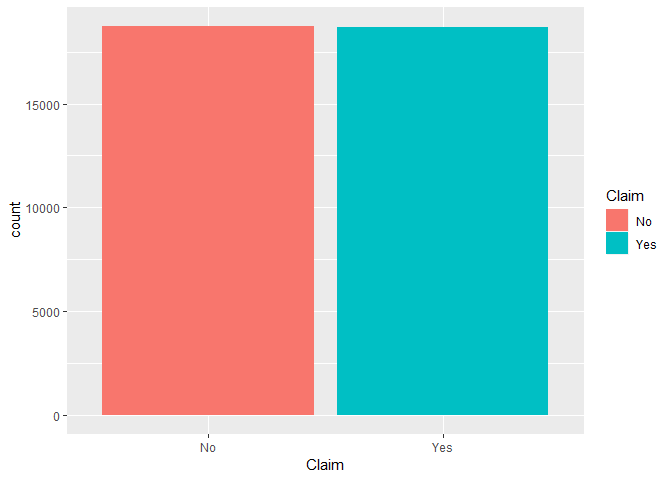### Random forest Data separation: sample <- sample.int(n = nrow(travel_oversampling), size = floor(0.7*nrow(travel_oversampling)), replace = F) train_o <- travel_oversampling[sample, ] test_o <- travel_oversampling[-sample, ] Training the model: rfModel_over <- randomForest(Claim ~., data = train_o) test_pred_rf_o <- predict(rfModel_over, newdata = test_o) Confusion matrix: cm_rf <- table(test_pred_rf_o, test_o$Claim)
cm_rf
##
## test_pred_rf_o   No  Yes
##            No  4788  130
##            Yes  864 5444
report <- measures(cm_rf, "Random Forest. Oversampling", report)
##  "Accuracy:  0.911455549616961"
##  "Precision:  0.976677430929315"
##  "Recall:  0.976677430929315"
##  "F1 Score:  0.976677430929315"

## Bothsampling

travel_bothsampling <- ovun.sample(Claim~. , data= travel_insurance_final, method = "both",
p=0.5, seed = 1000)$data ggplot(travel_bothsampling,aes(x=Claim, fill= Claim)) + geom_bar()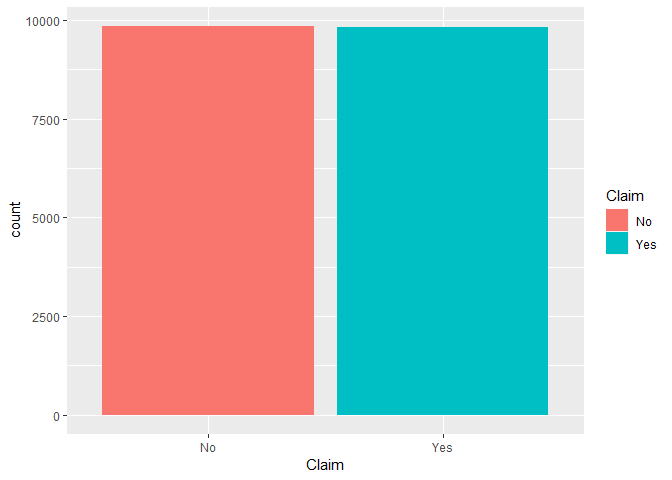### Random forest Data separation: sample <- sample.int(n = nrow(travel_bothsampling), size = floor(0.7*nrow(travel_bothsampling)), replace = F) train_b <- travel_bothsampling[sample, ] test_b <- travel_bothsampling[-sample, ] Training the model: rfModel_both <- randomForest(Claim ~., data = train_b) test_pred_rf_b <- predict(rfModel_both, newdata = test_b) Confusion matrix: cm_rf <- table(test_pred_rf_b, test_b$Claim)
cm_rf
##
## test_pred_rf_b   No  Yes
##            No  2553  120
##            Yes  360 2865
report <- measures(cm_rf, "Random Forest. Bothsampling", report)
##  "Accuracy:  0.918616480162767"
##  "Precision:  0.959798994974874"
##  "Recall:  0.959798994974874"
##  "F1 Score:  0.959798994974874"
report
##                    description  accuracy  precision     recall         f1
## 1                Random Forest 0.9526958 0.01805054 0.01805054 0.01805054
## 2 Random Forest. Undersampling 0.7356115 0.75268817 0.75268817 0.75268817
## 3  Random Forest. Oversampling 0.9114555 0.97667743 0.97667743 0.97667743
## 4  Random Forest. Bothsampling 0.9186165 0.95979899 0.95979899 0.95979899

## Cross Validation

Let’s perform Cross Validation for over- and both- sampled data.

For oversampled data:

cv_o_model<- rf.crossValidation(rfModel_over, train_o[-5], n=10)
## running: classification cross-validation with 10 iterations
 # Plot cross validation verses model producers accuracy
par(mfrow=c(1,2))
plot(cv_o_model, type = "cv", main = "CV", ylab = "accuracy")
plot(cv_o_model, type = "model", main = "Model without CV", ylab = "accuracy")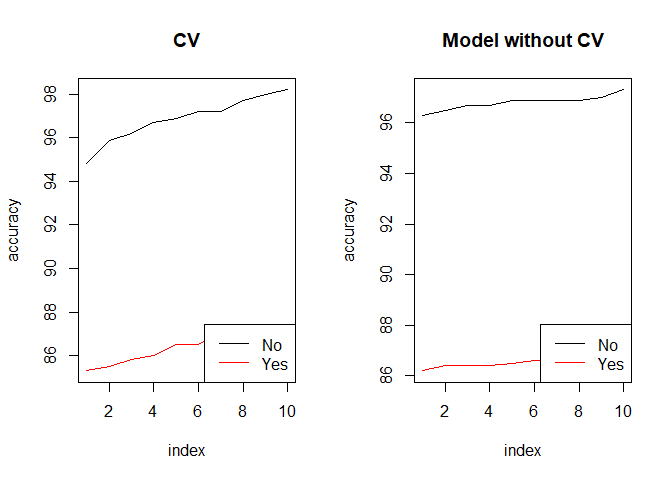# Plot cross validation verses model oob
par(mfrow=c(1,2))
plot(cv_o_model, type = "cv", stat = "oob", main = "CV oob", ylab = "error")
plot(cv_o_model, type = "model", stat = "oob", main = "Model without CV oob", ylab = "error")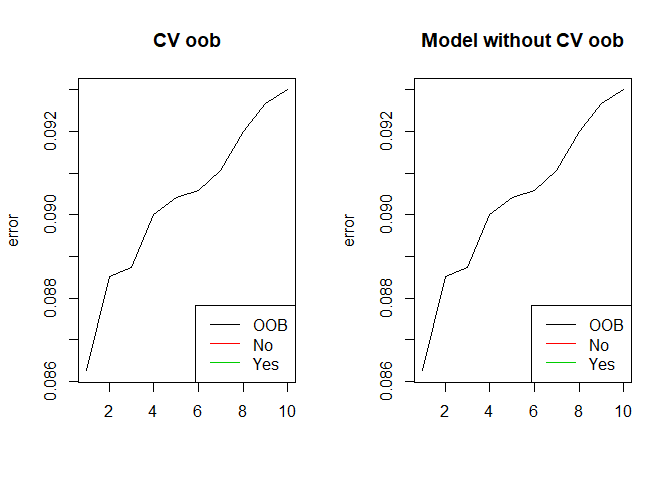For bothsampled data:

cv_b_model<- rf.crossValidation(rfModel_both, train_b[-5], n=10)
## running: classification cross-validation with 10 iterations
# Plot cross validation verses model producers accuracy
par(mfrow=c(1,2))
plot(cv_b_model, type = "cv", main = "CV", ylab = "accuracy")
plot(cv_b_model, type = "model", main = "Model", ylab = "accuracy")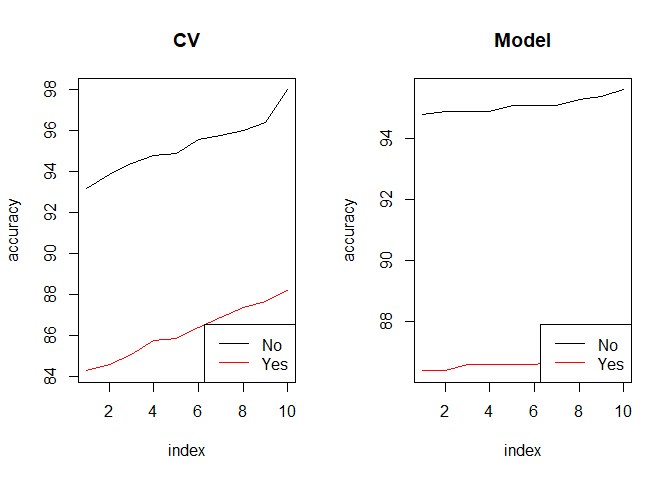# Plot cross validation verses model oob
par(mfrow=c(1,2))
plot(cv_b_model, type = "cv", stat = "oob", main = "CV oob", ylab = "error")
plot(cv_b_model, type = "model", stat = "oob", main = "Model oob", ylab = "error")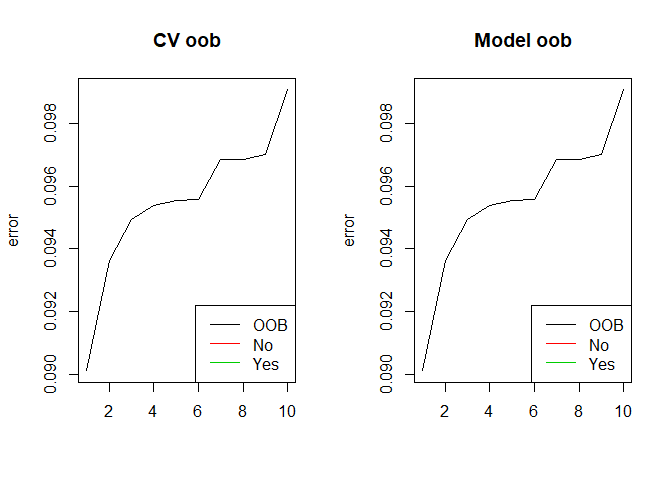# ROC & AUC

Let’s calculate ROC and AUC for predicted values:

roc_b <- roc(as.numeric(test_b$Claim), as.numeric(test_pred_rf_b)) # for bothsampled data auc(roc_b)  ## Area under the curve: 0.9181 roc_o <- roc(as.numeric(test_o$Claim), as.numeric(test_pred_rf_o)) # for oversampled data
auc(roc_o)
## Area under the curve: 0.9119
roc_u <- roc(as.numeric(test_u\$Claim), as.numeric(test_pred_rf_u)) # for undersampled data
auc(roc_u)
## Area under the curve: 0.7355

And plot all curves:

plot.roc(roc_b) # black - bothsampled
text(0.36, 0.53, labels=sprintf("AUC(B)): %0.3f", auc(roc_b)), col="black")

lines(roc_u, col="red", type='b')
text(0.36, 0.33, labels=sprintf("AUC(U)): %0.3f", auc(roc_u)), col="red") # red - undersampled

lines(roc_o, col="green", type='b')
text(0.36, 0.43, labels=sprintf("AUC(O): %0.3f", auc(roc_o)), col="green") # green - oversampled